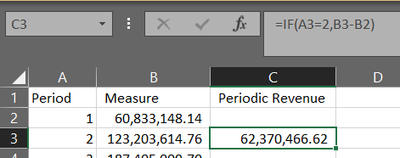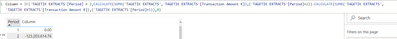cancel
Showing results for
Did you mean:Regular Visitor

## Un-accumulate a set values

I have a set of finance data that I have already had to transform and combine in Power Query, including applying exchange rates.

The values are accumulated with each Period. So P1 = P1, P2 = P1+P2. I now want to un-do that and just have P2 so P2-P1. I have looked at various online help articles but I am getting nowhere! I think I am confusing Measues and Columns as well which isn't helping and I don't understand the limitations of either/how they work.

I can do it in Excel so easily so I feel like this should be simple in PBI.

Excel:In PBI:Column = IF('TAGETIK EXTRACTS'[Period] = 2,CALCULATE(SUMX('TAGETIK EXTRACTS','TAGETIK EXTRACTS'[Transaction Amount €]),('TAGETIK EXTRACTS'[Period]=2))-CALCULATE(SUMX('TAGETIK EXTRACTS','TAGETIK EXTRACTS'[Transaction Amount €]),('TAGETIK EXTRACTS'[Period]=1)),0)

If that worked I was then going to expand it e.g.
Column = IF('TAGETIK EXTRACTS'[Period] = 2,CALCULATE(SUMX('TAGETIK EXTRACTS','TAGETIK EXTRACTS'[Transaction Amount €]),('TAGETIK EXTRACTS'[Period]=2))-CALCULATE(SUMX('TAGETIK EXTRACTS','TAGETIK EXTRACTS'[Transaction Amount €]),('TAGETIK EXTRACTS'[Period]=1))
IF('TAGETIK EXTRACTS'[Period] = 3,CALCULATE(SUMX('TAGETIK EXTRACTS','TAGETIK EXTRACTS'[Transaction Amount €]),('TAGETIK EXTRACTS'[Period]=3))-CALCULATE(SUMX('TAGETIK EXTRACTS','TAGETIK EXTRACTS'[Transaction Amount €]),('TAGETIK EXTRACTS'[Period]=2)),0)

Thanks,
5 REPLIES 5Regular Visitor

Apologies, I have multiple sets of periods. e.g. 2021 Actual, 2021 Budget, 2022 Actual, 2022 Budget etc. Even though on the current page I have a Filter so there is only 1 of each period.

Also, sorry I should have said before, the screenshots are not the raw data, they're already 'grouped'. The raw data is more like the below, so multiple entries for 1, 2 etc.

I did as you suggested above and only P1 is correct, I am assuming that's something to do with this?

Raw data structure example (simplified):

 File Period Department Type Amount Budget 1 A Revenue 1 Budget 1 B Cost 2 Budget 2 A Revenue 3 Budget 2 B Cost 4 Budget 3 A Revenue 6 Budget 3 B Cost 7 Budget 4 A Revenue 10 Budget 4 B Cost 11 Actual 1 A Revenue 1 Actual 1 B Cost 2 Actual 2 A Revenue 3 Actual 2 B Cost 4 Actual 3 A Revenue 6 Actual 3 B Cost 7 Actual 4 A Revenue 10 Actual 4 B Cost 11

Desired result (example of 1 sub group):

 File Period Department Type Amount Amount per Period Budget 1 B Cost 2 2 Budget 2 B Cost 4 2 Budget 3 B Cost 7 3 Budget 4 B Cost 11 4

So Amount per Period is the value I am trying to create.Super User

Sure, this works for the data you posted, is the real data much more complicated?

``````Amount per Period =
var _period = 'Table'[Period]

var _previous =
CALCULATE(
MAX('Table'[Amount]),
'Table'[Period] < _period,
ALL('Table'[Amount])
)

return 'Table'[Amount] - _previous``````Regular Visitor

Thanks. I can also get this to work for my test data:But unfortunately it isn't working for my actual dataset. When adding the new column and Period to a table, it still shows the accumulated values i.e. the same as the SUM of (my equivalent of) Test[Amount]? There are many more columns and rows of data in the real data as opposed to my example, but unfortunately I can't share it.

My real data contains negative values, but I just did the above test data with negative values and it was OK so I don't think it can be that.Super User

Ok, so for more columns can you give this a try?

``````Amount per Period =
var _period = 'Table'[Period]

var _previous =
CALCULATE(
MAX('Table'[Amount]),
'Table'[Period] < _period,
ALLEXCEPT('Table','Table'[File],'Table'[Department],'Table'[Type])
)

return 'Table'[Amount] - _previous``````

The columns inside the ALLEXCEPT need to be the ones you're looking to group by.Super User

You could add a custom column

``````Periodic Revenue =
'Table'[Measure] - MAXX(FILTER('Table', 'Table'[Period] < EARLIER('Table'[Period])), 'Table'[Measure])``````

Where Table is your table name and Period and Measure are the columns from your screenshot

This will work if you only have one year's data (so one set of periods 1 through 12)Announcements#### Exclusive opportunity for Women!

Join us for a free, hands-on Microsoft workshop led by women trainers for women where you will learn how to build a Dashboard in a Day!#### Power Platform Conference-Power BI and Fabric Sessions

Join us Oct 1 - 6 in Las Vegas for the Microsoft Power Platform Conference.Top Solution Authors
Top Kudoed Authors
Users online (3,757)